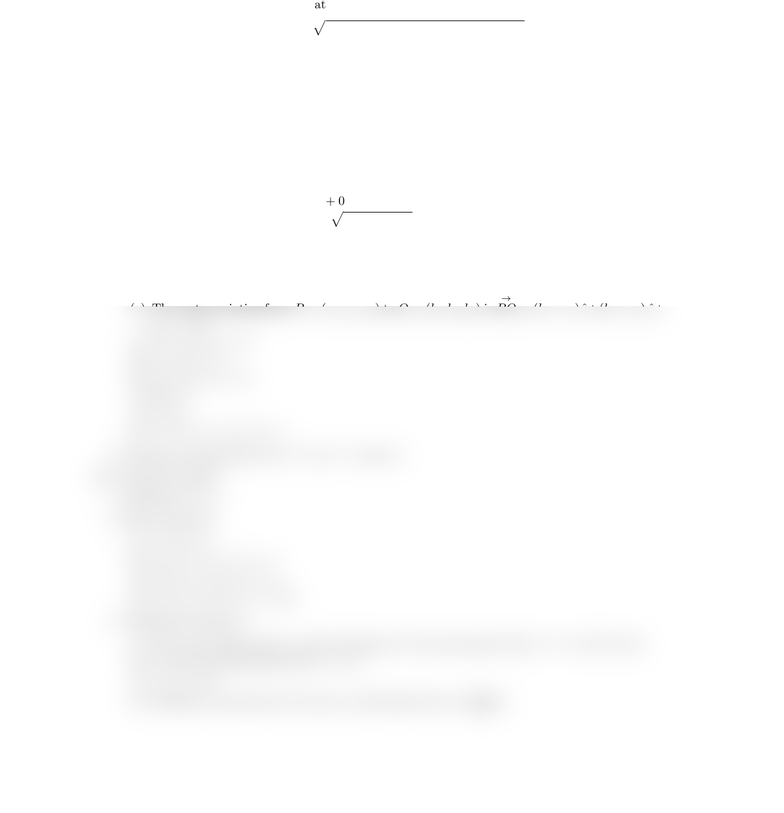# CSCI 2170 Lecture Notes - Lecture 11: Unit Vector, Global Positioning System

21 views2 pages
School
Course
ProfessorMath 241 Chapter 11 Dr. Justin O. Wyss-Gallifent
§11.1 Cartesian Coordinates in Space
1. Preliminaries: How to plot points in 3-space, the coordinate planes, the ﬁrst octant. Emphasize
how perspective can be confusing at ﬁrst.
2. Distance between points: |P Q|=p(x1x0)2+ (y1y0)2+ (z1z0)2
3. Equation of a circle, a closed disk, a sphere and a closed ball. Pictures of all.
§11.2 Vectors in Space
1. Deﬁnition of a vector as a triple of numbers. The notation ¯aor
a. We can add and subtract vectors
by adding and subtracting components and we can multiply a scalar by a vector by multiplying
by all the components. Three special vectors are ˆı= (1,0,0), ˆ= (0,1,0) and ˆ
k= (0,0,1).
Then every vector can be written as ¯a=a1ˆı+a2ˆ+a3ˆ
k. Vectors are not necessarily anchored
anywhere though often we anchor them somewhere (the origin, for example) for some reason.
2. Basic properties and associated deﬁnitions:
(a) The zero vector is ¯
0 = 0 ˆı+ 0 ˆ+ 0 ˆ
k.
(b) The length of a vector is ||¯a|| =pa2
1+a2
2+a2
3.
(c) A unit vector has length 1. If a vector ¯ais given we can create a unit vector in the same
direction by doing ¯a/||¯a||.
(d) Two vectors are parallel if they are nonzero multiple of one another. In other words ¯a=c¯
b
with c6= 0.
(e) The vector pointing from P= (a1, a2, a3) to Q= (b1, b2, b3) is
P Q = (b1a1) ˆı+(b2a2) ˆ+
(b3a3)ˆ
k.
(f) ¯
0 + ¯a= ¯a= ¯a+¯
0
(g) ¯a+¯
b=¯
b+ ¯a
(h) c(¯a+¯
b) = c¯a+c¯
b
(i) 0¯a=¯
0
(j) 1¯a= ¯a
(k) ¯a+ (¯
b+ ¯c) = (¯a+¯
b) + ¯c
3. Geometric interpretation of ¯a+¯
b, of ¯a¯
band c¯a.
§11.3 The Dot Product
1. Denition of ¯a·¯
b.
2. Basic properties:
(a) ¯a·¯
b=¯
b·¯a
(b) ¯a·(¯
b+ ¯c) = ¯a·¯
b+ ¯a·¯c
(c) (¯
b+ ¯c)·¯a=¯
b·¯a+ ¯c·¯a
(d) c(¯a·¯
b) = (c¯a)·¯
b= ¯a·(c¯
b)
(a) If θis the angle between ¯aand ¯
b(anchored at the same point) then ¯a·¯
b=||¯a||||¯
b|| cos θ.
(b) ¯aand ¯
bare perpendicular i ¯a·¯
b= 0.
(c) ¯a·¯a=||¯a||2.
(d) Denition of projection of ¯
bonto ¯aand formula Pr¯a¯
b=¯a·¯
b
¯a·¯a¯a.
Unlock document

This preview shows half of the first page of the document.
Unlock all 2 pages and 3 million more documents.